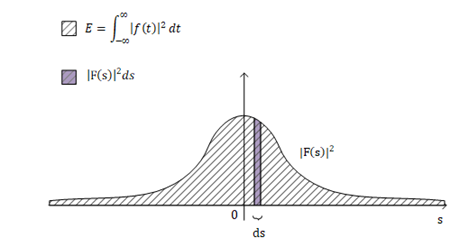用三角函数表示周期函数

$f(t) = \frac{a_0}{2}+\displaystyle{\sum^{\infty}_{k=1}}(a_kcos(2\pi kt)+b_ksin(2\pi kt))$

傅里叶级数

$f(t) = \displaystyle{\sum^{\infty}_{k=-\infty}C_ke^{2\pi ikt} }$

$C_k = \displaystyle{\int_{0}^{1}f(t)e^{-2\pi ikt}dt}$

傅里叶变换

$f(t) = \displaystyle{\lim_{T\to\infty}\sum_{k=-\infty}^{\infty}C_ke^{2\pi i\frac{k}{T}t} }$

$C_k = \displaystyle{\lim_{T\to\infty}\frac{1}{T}\int_{-\frac{T}{2}}^{\frac{T}{2}}f(t)e^{-2\pi i\frac{k}{T}t}dt }$

$\displaystyle{\mathcal{F} f(s) =C_k \times T = \int_{-\infty}^{\infty}e^{-2\pi i st}f(t)dt }$

$f(t) = \displaystyle{\int_{-\infty}^{\infty}\mathcal{F} f(s)e^{2\pi ist}ds }$

$\displaystyle{\mathcal{F} f(s) }$就是信号$f(t)$的傅里叶变换。但此时傅里叶变换不再具有傅里叶系数的物理意义。

傅里叶变换的物理意义

Plancherel's Formula

Plancherel's Formula有如下定义：

$\displaystyle{\int_{-\infty}^{\infty}f(t)\overline{g(t)}\,dt=\int_{-\infty}^{\infty}F(s)\overline{G(s)}\,ds}$

\begin{align*}\int_{-\infty}^{\infty}f(t)\overline{g(t)}\,dt &=\int_{-\infty}^{\infty}\left(\int_{-\infty}^{\infty}F(s)e^{2\pi ist}\,ds \right )\overline{\left(\int_{-\infty}^{\infty}G(s')e^{2\pi is't}\,ds' \right )}\,dt \\ &=\int_{-\infty}^{\infty}\left(\int_{-\infty}^{\infty}F(s)e^{2\pi ist}\,ds \right )\left(\int_{-\infty}^{\infty}\overline{G(s')}e^{-2\pi is't}\,ds' \right )\,dt \\ &=\int_{-\infty}^{\infty}\int_{-\infty}^{\infty}F(s)\overline{G(s')}\int_{-\infty}^{\infty}e^{2\pi i(s-s')t}\,dt\,ds'\,ds \\ &=\int_{-\infty}^{\infty}\int_{-\infty}^{\infty}F(s)\overline{G(s')}\delta(s'-s)\,ds'\,ds\qquad \mathcal{F}e^{2\pi ist} = \delta(s'-s)\ ,variable\ is\ s'\\ &=\int_{-\infty}^{\infty}F(s)\int_{-\infty}^{\infty}\overline{G(s')}\delta(s'-s)\,ds'\,\,ds \\ &=\int_{-\infty}^{\infty}F(s)\,\overline{G(s)}\,ds \qquad \delta\ shift\ theorem\\ \end{align*}

Energy Spectral Density

\begin{align*} \int_{-\infty}^{\infty}|f(t)|^2\,dt &=\int_{-\infty}^{\infty}f(t)\overline{f(t)}\,dt\\ &=\int_{-\infty}^{\infty}F(s)\overline{F(s)}\,ds\\ &=\int_{-\infty}^{\infty}|F(s)|^2\,ds \end{align*}

$\displaystyle{\int_{-\infty}^{\infty}\frac{V(t)^2}{R}dt}$

$\displaystyle{\int_{-\infty}^{\infty}|f(t)|^2\,dt=\int_{-\infty}^{\infty}|F(s)|^2\,ds}$posted @ 2019-01-08 02:39  TaigaComplex  阅读(7410)  评论(0编辑  收藏  举报